If you're running AdBlock, please consider whitelisting this site if you'd like to support LearnOpenGL; and no worries, I won't be mad if you don't :)

# Collision detection

When trying to determine if a collision occurs between two objects, we generally do not use the data of the objects themselves since these objects are often quite complicated; this in turn also makes the collision detection complicated. For this reason, it is a common practice to use more simple shapes (that usually have a nice mathematical definition) for collision detection that we overlay on top of the original object. We then check for collisions based on these simple shapes which makes the code easier and saves a lot of performance. Several examples of such collision shapes are circles, spheres, rectangles and boxes; these are a lot simpler to work with compared to meshes with hundreds of triangles.

While they do give us easier and more efficient collision detection algorithms, the simpler collision shapes share a common disadvantage in that these shapes usually do not fully surround the object. The effect is that a collision might be detected that didn't really collide with the actual object; one should always keep in mind that these shapes are just approximations of the real shapes.

## AABB - AABB collisions

AABB stands for axis-aligned bounding box which is a rectangular collision shape aligned to the base axes of the scene, which in 2D aligns to the x and y axis. Being axis-aligned means the rectangular box is not rotated and its edges are parallel to the base axes of the scene (e.g. left and right edge are parallel to the y axis). The fact that these boxes are always aligned to the axes of the scene makes all calculations easier. Here we surround the ball object with an AABB: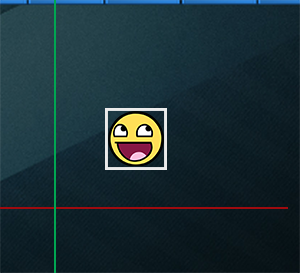Almost all the objects in Breakout are rectangular based objects so it makes perfect sense to use axis aligned bounding boxes for detecting collisions. This is exactly what we're going to do.

Axis aligned bounding boxes can be defined in several ways. One of the ways to define an AABB is by having a top-left position and a bottom-right position. The GameObject class that we defined already contains a top-left position (its Position vector) and we can easily calculate its bottom-right position by adding its size to the top-left position vector (Position + Size). Effectively, each GameObject contains an AABB that we can use for collisions.

So how do we determine collisions? A collision occurs when two collision shapes enter each other's regions e.g. the shape that determines the first object is in some way inside the shape of the second object. For AABBs this is quite easy to determine due to the fact that they're aligned to the scene's axes: we check for each axis if the two object' edges on that axis overlap. So basically we check if the horizontal edges overlap and if the vertical edges overlap of both objects. If both the horizontal and vertical edges overlap we have a collision.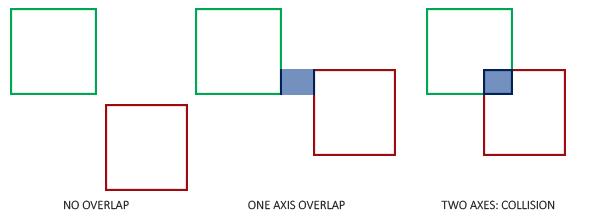Translating this concept to code is quite straightforward. We check for overlap on both axes and if so, return a collision:


GLboolean CheckCollision(GameObject &one, GameObject &two) // AABB - AABB collision
{
// Collision x-axis?
bool collisionX = one.Position.x + one.Size.x >= two.Position.x &&
two.Position.x + two.Size.x >= one.Position.x;
// Collision y-axis?
bool collisionY = one.Position.y + one.Size.y >= two.Position.y &&
two.Position.y + two.Size.y >= one.Position.y;
// Collision only if on both axes
return collisionX && collisionY;
}


We check if the right side of the first object is greater than the left side of the second object and if the second object's right side is greater than the first object's left side; similarly for the vertical axis. If you have trouble visualizing this, try to draw the edges/rectangles on paper and determine this for yourself.

To keep the collision code a bit more organized we add an extra function to the Game class:


class Game
{
public:
[...]
void DoCollisions();
};


Within DoCollisions we check for collisions between the ball object and each brick of the level. If we detect a collision, we set the brick's Destroyed property to true which also instantly stops the level from rendering this brick.


void Game::DoCollisions()
{
for (GameObject &box : this->Levels[this->Level].Bricks)
{
if (!box.Destroyed)
{
if (CheckCollision(*Ball, box))
{
if (!box.IsSolid)
box.Destroyed = GL_TRUE;
}
}
}
}


Then we also need to update the game's Update function:


void Game::Update(GLfloat dt)
{
// Update objects
Ball->Move(dt, this->Width);
// Check for collisions
this->DoCollisions();
}


If we run the code now, the ball should detect collisions with each of the bricks and if the brick is not solid, the brick is destroyed. If you run the game now it'll look something like this:

While the collision detection does work, it's not very precise since the ball collides with most of the bricks without directly touching them. Let's implement another collision detection technique.

## AABB - Circle collision detection

Beacuse the ball is a circle-like object an AABB is probably not the best choice as the ball's collision shape. The collision code thinks the ball is a rectangular box so the ball often collides with a brick even though the ball sprite itself isn't yet touching the brick.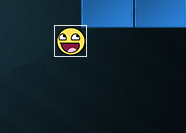It makes much more sense to represent the ball with a circle collision shape instead of an AABB. For this reason we included a Radius variable within the ball object. To define a circle collision shape all we need is a position vector and a radius.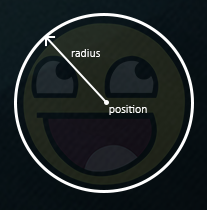This does mean we have to update the detection algorithm since it currently only works between two AABBs. Detecting collisions between a circle and a rectangle is slightly more complicated, but the trick is as follows: we find the point on the AABB that is closest to the circle and if the distance from the circle to this point is less than its radius, we have a collision.

The difficult part is getting this closest point $$\color{red}{\bar{P}}$$ on the AABB. The following image shows how we can calculate this point for any arbitrary AABB and circle: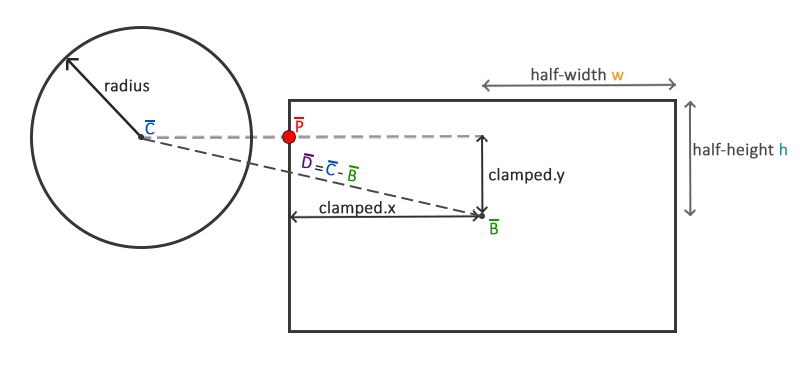We first need to get the difference vector between the ball's center $$\color{blue}{\bar{C}}$$ and the AABB's center $$\color{green}{\bar{B}}$$ to obtain $$\color{purple}{\bar{D}}$$. What we then need to do is clamp this vector $$\color{purple}{\bar{D}}$$ to the AABB's half-extents $$\color{orange}{{w}}$$ and $$\color{teal}{\bar{h}}$$. The half-extents of a rectangle are the distances between the rectangle's center and its edges; basically its size divided by two. This returns a position vector that is always located somewhere at the edge of the AABB (unless the circle's center is inside the AABB).

A clamp operation clamps a value to a value within a given range. This is often expressed as:

float clamp(float value, float min, float max) {
return std::max(min, std::min(max, value));
}

For example, a value of 42.0f is clamped as 6.0f between 3.0f and 6.0f and a value of 4.20f would be clamped to 4.20f.
Clamping a 2D vector means we clamp both its x and its y component within the given range.

This clamped vector $$\color{red}{\bar{P}}$$ is then the closest point from the AABB to the circle. What we then need to do is calculate a new difference vector $$\color{purple}{\bar{D'}}$$ that is the difference between the circle's center $$\color{blue}{\bar{C}}$$ and the vector $$\color{red}{\bar{P}}$$.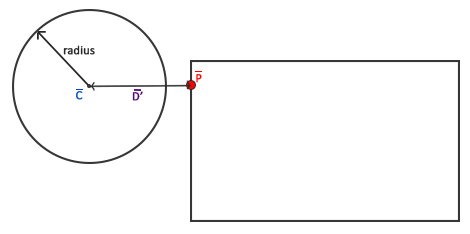Now that we have the vector $$\color{purple}{\bar{D'}}$$ we can compare its length to the radius of the circle to determine if we have a collision.

This is all expressed in code as follows:


GLboolean CheckCollision(BallObject &one, GameObject &two) // AABB - Circle collision
{
// Get center point circle first
// Calculate AABB info (center, half-extents)
glm::vec2 aabb_half_extents(two.Size.x / 2, two.Size.y / 2);
glm::vec2 aabb_center(
two.Position.x + aabb_half_extents.x,
two.Position.y + aabb_half_extents.y
);
// Get difference vector between both centers
glm::vec2 difference = center - aabb_center;
glm::vec2 clamped = glm::clamp(difference, -aabb_half_extents, aabb_half_extents);
// Add clamped value to AABB_center and we get the value of box closest to circle
glm::vec2 closest = aabb_center + clamped;
// Retrieve vector between center circle and closest point AABB and check if length <= radius
difference = closest - center;

Using these collision shape attributes we calculate vector $$\color{purple}{\bar{D}}$$ as difference that we then clamp to clamped and add to the AABB's center to get point $$\color{red}{\bar{P}}$$ as closest. Then we calculate the difference vector $$\color{purple}{\bar{D'}}$$ between center and closest and return whether the two shapes collided or not.